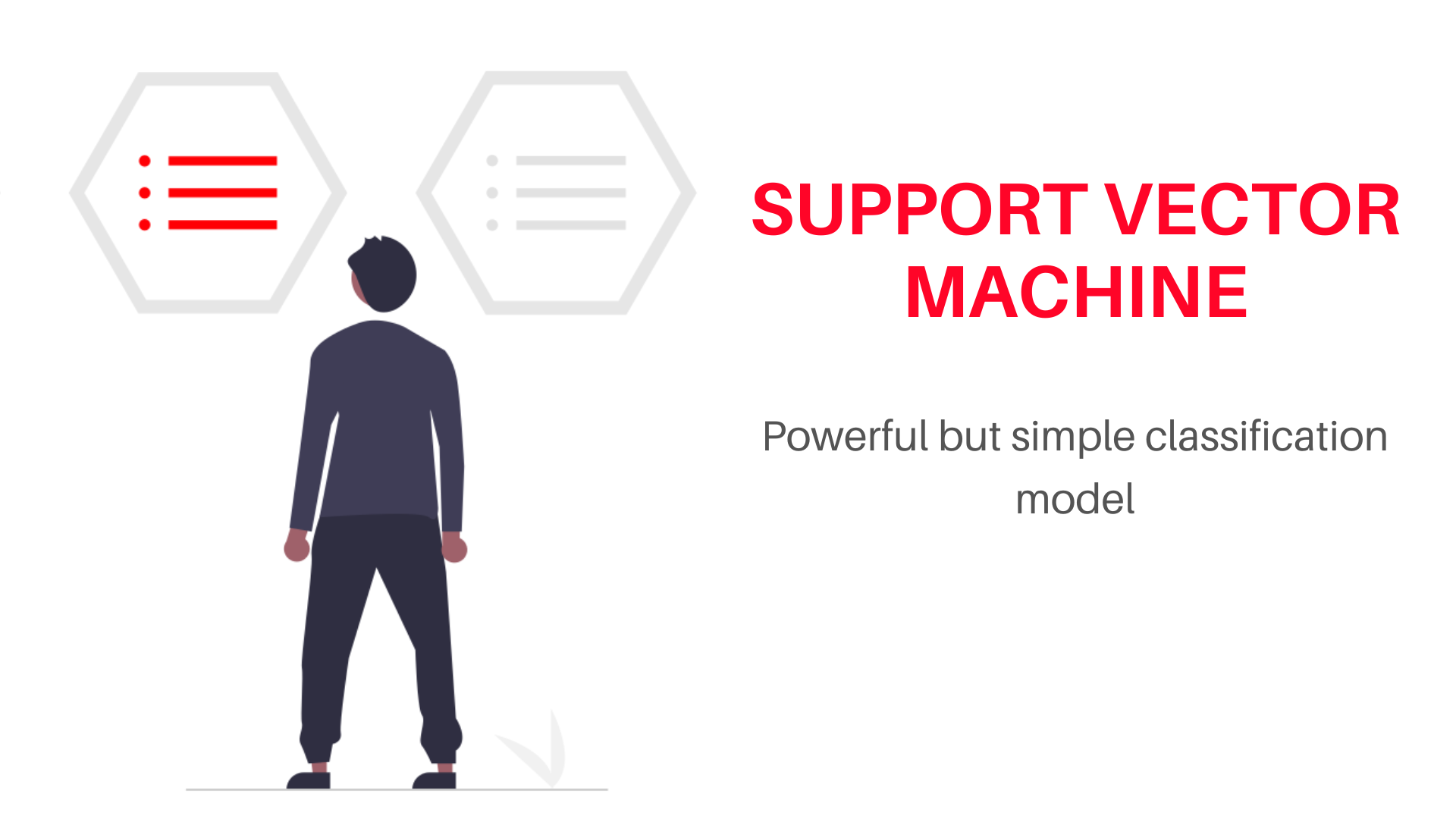# Support Vector Machine: One of the easiest classification modelToday, let’s discuss Support Vector Machine, or SVM for short. SVM can be used for both regressions as well as classification. Let’s see how SVM looks like a graph in both cases.

By looking at these two graphs you should have got an idea that SVM is not working well in Regression but it is working well enough in the case of classification. What you are looking for in a classification graph is called Binary classification where there are only 2 categories in the target.

Let’s discuss what are those keywords given to different parts of SVM.

Support Vectors:- The data point that is closer to the hyperplane.

Margin:- It is a line that is away from the decision boundary.

Decision Boundary or Hyperplane:- It is the main dividing line that divides two classes.

When you work with the real dataset, you will not get to see such a dataset that you are looking at here in the case of classification. You are lucky if you get such data because, in real datasets, the data will be very complex and cannot be divided easily.

Let us discuss some of the hyperparameters that you will be working on

## Regularization in support vector machine

C Is The Regularization Parameter Using Which We Can Decrease The Model Complexity Which In Turn Avoid Overfitting Our Model. In other more simple Words, the C Parameter Helps the Model To Classify The Data More Precisely. Let’s Visualize This To Understand Better.

When C = 1, the margins are bigger as compared to when C = 10, and you can also observe that when margins are bigger then the chances of misclassification is also high and in the case of c = 10 (lower margins), the misclassification has not happened.

Misclassification:- It is the case when the model predicts class Red as class Green and vice-versa

But in reality, there will be misclassification of data for sure else the model will be overfitted.

Also, read -> what is regularization and how it works

## Gamma in support vector machine

What if the data is not well separated and you cannot apply linear Kernel ( a linear line that will separate the two classes like in the above diagrams). You need to use other kernels like Poly or RBF, and with those kernels, you need to use gamma. So this will help you to separate the classes.

Let’s understand this with graphs.

This data cannot be divided linearly so you need to use different kernels like Poly or RBF

Look at the hyperplane, it has well divided the data but still, there are lots of misclassification, to solve this we can increase the gamma value. You see the red background and green background, whatever the data falls inside the red background is predicted as class red and whatever the data has fallen inside the green background is predicted as class green.

Now increasing the gamma value has improved the result

## kernelin Support vector machine

When we want to apply SVM on non-linearly separable data we apply non-linear kernels available like Poly and RBF. Here comes the Kernel trick, when non-linearly separable data is passed to the model, the Low Dimensional data which is not linearly separable is converted to High Dimensional Data which is now Linearly separable.

Look at the below given first graph, it cannot be classified using the linear kernel, so we will apply the Kernel trick here.

#### 1) Polynomial Kernel

This is applied to data that is not linearly separable. When you use the poly kernel, you also tune a hyperparameter called a degree. The degree allows flexibility to the polynomial kernel. If degree = 1, then it results in a linear kernel, higher degree results in a more flexible kernel. Let’s look at some graphs to understand this.

#### RBF Kernel

Radial Basis Function or RBF for short is my favorite as it works very well in case of data that is not linearly separable. Let me show some of its graphs to explain to you why it is my favorite

You can observe that on increasing the gamma value, the model is able to classify the data very well. But one thing you need to keep in mind is that increasing the gamma value can lead to overfitting.

## Soft Margin and Hard Margin in SVM

#### Hard Margin

The core idea of Hard Margin is to maximize the margin in a way that classifier does not make any mistake i.e. it does not misclassify the data. But this is problematic because it becomes very sensitive to new data and tries to fit the decision boundary according to that new data.

You can observe that to satisfy new data and to avoid any misclassification, the decision boundary in the case of Hard Margin got shifted.

#### Soft Margin

The problem of Hard Margin is overcome by Soft margin. Soft Margin allows some misclassification, so countering any new data will not shift its decision boundary.

Observe this, the decision boundary is not shifted at all. The benefit of a soft margin is that it can avoid overfitting.

Some points to remember when using SVM…..

Can be used for both Regression and Classification but does not work well with regression.

Can be used when lots of outliers are present.

But remember that SVM is slower to train and its prediction speed is Moderate.

It gives high performance when the data is small.

https://en.wikipedia.org/wiki/Support-vector_machine

https://scikit-learn.org/stable/modules/generated/sklearn.svm.SVC.html##### Aman Kumar

Data Scientist with 3+ years of experience in building data-intensive applications in diverse industries. Proficient in predictive modeling, computer vision, natural language processing, data visualization etc. Aside from being a data scientist, I am also a blogger and photographer.